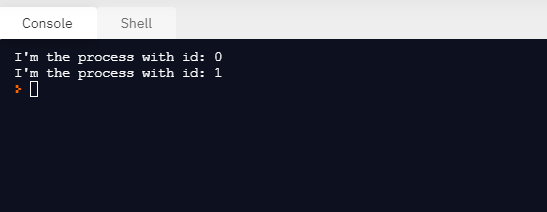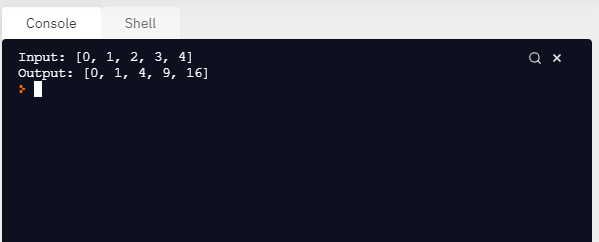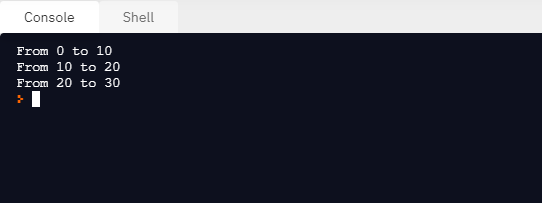# Python – Run same function in parallel with different parameters

• Difficulty Level : Medium
• Last Updated : 03 Mar, 2021

In this article, we will learn how to run the same function in parallel with different parameters. We can run the same function in parallel with different parameters using parallel processing.

The number of tasks performed by the program can be increased by parallel processing, which decreases the total processing time. These assist in addressing large-scale issues. Using the standard multiprocessing module, by creating child processes, we can effectively parallelize simple tasks. This module provides an easy-to-use interface and includes a set of task submission and synchronization handling utilities.

Approach:

• We may construct a process running independently by subclassing the multiprocessing process. We can initialize the resource by extending the __init_ method, and we can write the code for the subprocess by implementing the Process.run() method. We see how to construct a process in the code below, which prints the assigned id.
• We need to initialize our process object and invoke the Process.start() method in order to spawn the process. Here, Process.start() will create a new process and invoke a method called Process.run().
• The code after p.start() is executed immediately before process p completes the mission. You may use Process.join to wait for task completion().

Let’s understand this with some examples.

Example 1:

## Python3

 `import` `multiprocessing``import` `time`` ` `# Process class``class` `Process(multiprocessing.Process):``    ``def` `__init__(``self``, ``id``):``        ``super``(Process, ``self``).__init__()``        ``self``.``id` `=` `id`` ` `    ``def` `run(``self``):``        ``time.sleep(``1``)``        ``print``(``"I'm the process with id: {}"``.``format``(``self``.``id``))`` ` ` ` `if` `__name__ ``=``=` `'__main__'``:``    ``p ``=` `Process(``0``)`` ` `    ``# Create a new process and invoke the``    ``# Process.run() method``    ``p.start()`` ` `    ``# Process.join() to wait for task completion.``    ``p.join()``    ``p ``=` `Process(``1``)``    ``p.start()``    ``p.join()`

Output:We can also run the same function in parallel with different parameters using the Pool class. For parallel mapping, We have to first initialize multiprocessing.Pool() object. The first argument is the number of workers; if not given, that number will be equal to the number of elements in the system.

Example 2:

Let see by an example. In this example, we will see how to pass a function that computes the square of a number. Using Pool.map() we can map the function to the list and passing the function and the list of inputs as arguments, as follows:

## Python3

 `import` `multiprocessing``import` `time`` ` `# square function``def` `square(x):``    ``return` `x ``*` `x`` ` ` ` `if` `__name__ ``=``=` `'__main__'``:`` ` `    ``# multiprocessing pool object``    ``pool ``=` `multiprocessing.Pool()`` ` `    ``# pool object with number of element``    ``pool ``=` `multiprocessing.Pool(processes``=``4``)`` ` `    ``# input list``    ``inputs ``=` `[``0``, ``1``, ``2``, ``3``, ``4``]`` ` `    ``# map the function to the list and pass``    ``# function and input list as arguments``    ``outputs ``=` `pool.``map``(square, inputs)`` ` `    ``# Print input list``    ``print``(``"Input: {}"``.``format``(inputs))`` ` `    ``# Print output list``    ``print``(``"Output: {}"``.``format``(outputs))`

Output:Example 3:

## Python3

 `from` `multiprocessing ``import` `Pool`` ` ` ` `def` `print_range(``range``):``   ` `    ``# print range``    ``print``(``'From {} to {}:'``.``format``(``range``[``0``], ``range``[``1``]))`` ` ` ` `def` `run_parallel():``   ` `    ``# list of ranges``    ``list_ranges ``=` `[[``0``, ``10``], [``10``, ``20``], [``20``, ``30``]]`` ` `    ``# pool object with number of elements in the list``    ``pool ``=` `Pool(processes``=``len``(list_ranges))`` ` `    ``# map the function to the list and pass ``    ``# function and list_ranges as arguments``    ``pool.``map``(print_range, list_ranges)`` ` `# Driver code``if` `__name__ ``=``=` `'__main__'``:``    ``run_parallel()`

Output:My Personal Notes arrow_drop_up